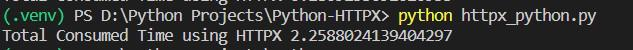Open in App
Not now

# Increase the speed of Web Scraping in Python using HTTPX module

• Last Updated : 23 Jan, 2023

In this article, we will talk about how to speed up web scraping using the requests module with the help of the HTTPX module and AsyncIO by fetching the requests concurrently.

The user must be familiar with Python. Knowledge about the Requests module or web scraping would be a bonus.

#### Required Module

For this tutorial, we will use 4 modules –

• time
• requests
• httpx
• asyncio.
`pip install httpx`
`pip install requests`

time and asyncio comes pre-installed so no need to install them.

### Using the requests module to get the required time –

First, we will use the traditional way of fetching URLs using the get() method of the requests module, then using the time module we will check the total time consumed.

## Python3

 `import` `time``import` `requests`` ` ` ` `def` `fetch_urls():``    ``urls``=``[``        ``"https://en.wikipedia.org/wiki/Badlands"``,``        ``"https://en.wikipedia.org/wiki/Canyon"``,``        ``"https://en.wikipedia.org/wiki/Cave"``,``        ``"https://en.wikipedia.org/wiki/Cliff"``,``        ``"https://en.wikipedia.org/wiki/Coast"``,``        ``"https://en.wikipedia.org/wiki/Continent"``,``        ``"https://en.wikipedia.org/wiki/Coral_reef"``,``        ``"https://en.wikipedia.org/wiki/Desert"``,``        ``"https://en.wikipedia.org/wiki/Forest"``,``        ``"https://en.wikipedia.org/wiki/Geyser"``,``        ``"https://en.wikipedia.org/wiki/Mountain_range"``,``        ``"https://en.wikipedia.org/wiki/Peninsula"``,``        ``"https://en.wikipedia.org/wiki/Ridge"``,``        ``"https://en.wikipedia.org/wiki/Savanna"``,``        ``"https://en.wikipedia.org/wiki/Shoal"``,``        ``"https://en.wikipedia.org/wiki/Steppe"``,``        ``"https://en.wikipedia.org/wiki/Tundra"``,``        ``"https://en.wikipedia.org/wiki/Valley"``,``        ``"https://en.wikipedia.org/wiki/Volcano"``,``        ``"https://en.wikipedia.org/wiki/Artificial_island"``,``        ``"https://en.wikipedia.org/wiki/Lake"``    ``]`` ` `    ``res ``=` `[requests.get(addr).status_code ``for` `addr ``in` `urls]`` ` `    ``print``(``set``(res))`` ` ` ` ` ` ` ` `start ``=` `time.time()``fetch_urls()``end ``=` `time.time()`` ` `print``(``"Total Consumed Time"``,end``-``start)`

Firstly we imported the requests and time module then created a function called fetch_urls() inside which we created a list consisting of 20 links (user can choose any number of any random links which exists). Then inside a variable res which is a type of list we are using the get() method with the status_code method of requests module to send a request to each of those links and fetch and store their status_codes as a list. Then lastly we are printing the set of that res. Now main reason of converting it into a set is that if everysite is working then all will return 200 status code so making it set will only be a single value so the time consumption will be less in that work (Motive is to use as less time as possible in other works).

Then outside the function using the time() method of time module we are storing the starting and ending time and in between calling the function. Then finally printing the total consumed time.

Output:We can see from the output that it consumed total 12.6422558 seconds.

## Python3

 `import` `time``import` `asyncio``import` `httpx`` ` ` ` ` ` `async ``def` `fetch_httpx():``    ``urls``=``[``        ``"https://en.wikipedia.org/wiki/Badlands"``,``        ``"https://en.wikipedia.org/wiki/Canyon"``,``        ``"https://en.wikipedia.org/wiki/Cave"``,``        ``"https://en.wikipedia.org/wiki/Cliff"``,``        ``"https://en.wikipedia.org/wiki/Coast"``,``        ``"https://en.wikipedia.org/wiki/Continent"``,``        ``"https://en.wikipedia.org/wiki/Coral_reef"``,``        ``"https://en.wikipedia.org/wiki/Desert"``,``        ``"https://en.wikipedia.org/wiki/Forest"``,``        ``"https://en.wikipedia.org/wiki/Geyser"``,``        ``"https://en.wikipedia.org/wiki/Mountain_range"``,``        ``"https://en.wikipedia.org/wiki/Peninsula"``,``        ``"https://en.wikipedia.org/wiki/Ridge"``,``        ``"https://en.wikipedia.org/wiki/Savanna"``,``        ``"https://en.wikipedia.org/wiki/Shoal"``,``        ``"https://en.wikipedia.org/wiki/Steppe"``,``        ``"https://en.wikipedia.org/wiki/Tundra"``,``        ``"https://en.wikipedia.org/wiki/Valley"``,``        ``"https://en.wikipedia.org/wiki/Volcano"``,``        ``"https://en.wikipedia.org/wiki/Artificial_island"``,``        ``"https://en.wikipedia.org/wiki/Lake"``    ``]`` ` `    ``async with httpx.AsyncClient() as httpx_client:``        ``req ``=` `[httpx_client.get(addr) ``for` `addr ``in` `urls]`` ` `        ``result ``=` `await asyncio.gather(``*``req)`` ` ` ` ` ` ` ` ` ` ` ` ` ` `start ``=` `time.time()``asyncio.run(fetch_httpx())``end ``=` `time.time()`` ` `print``(``"Total Consumed Time using HTTPX"``,end``-``start)`

we have to use asyncio with HTTPX otherwise we can’t send requests concurrently. HTTPX itself has an inbuilt AsyncIO client which we will use here, for using that inside a function the function has be to Asynchronous. We are calling the AsyncClient() method of HTTPX module using the alias httpx_client, then using that alias we are concurrently sending requests to the same links used earlier. Then as we are using async we have to use await, using the await we are gathering the response and storing them in result. (If user wants they can print that too, but as my intention is to decrease the time consumed in other operations rather than fetching requests I didn’t print them.).

Then from outside the function using the asyncio.run() method we are calling that function and then printing the result of the total time consumed.

#### Output:As we can see from the Output the total time consumed has been decrease by nearly 6 times. This difference can differ anytime. If we are sending requests to the same URL again and again then the time consumed for both requests and HTTPX will be lesser than last time so this difference will then be increased more.

Here the difference is nearly 10 times, HTTPX with AsyncIO is nearly 10 times faster than requests.

My Personal Notes arrow_drop_up